Ask your WordPress questions! Pay money and get answers fast!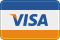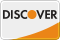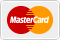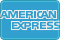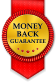# Total entries by author (entries being sum of posts and comments) WordPress

• SOLVED

with

`the_author_posts(); `

I can get total number of posts by author.

with

```function author_comment_count(){ \$oneText = '1'; \$moreText = '%'; global \$wpdb; \$result = \$wpdb->get_var(' SELECT COUNT(comment_ID) FROM '.\$wpdb->comments.' WHERE comment_author_email = "'.get_comment_author_email().'"' ); if(\$result == 1): echo str_replace('%', \$result, \$oneText); elseif(\$result > 1): echo str_replace('%', \$result, \$moreText); endif; } ```

I can get total number of comments by author.

But I'm unable to sum the 2 values.

I tried something like this:

``` <?php function tpc(){ \$comms = author_comment_count(); \$posts = the_author_posts(); \$total_c_p = \$comms + \$posts; return \$total_c_p; } ?> <?php tpc();?> ```

But with both \$comms and \$posts returning 1, the tpc function returns 11 instead of 2???

What am I doing wrong?

2011-07-30

You are echoing the values instead of returning them. Try get_the_author_posts() instead of the_author_posts().

``` if(\$result == 1): echo str_replace('%', \$result, \$oneText); elseif(\$result > 1): echo str_replace('%', \$result, \$moreText); endif;```

Just use
`return result;`

Now Add the values and show the result.

Hi Navjot Singh,

I think that's the right path, but now it returns 1 instead of 2.

I don't know why, but get_the_author_posts() is returning 0

Are you using the code inside the loop? No reason it should return 0.

Actually it's working...

it wasn't get_the_author_posts() to return 0, but author_comment_count(); returning "results"...

"return result;"

into

"return \$result;"

Try `\$total_c_p = (int)\$comms + (int)\$posts;`